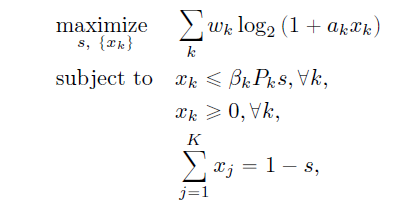# Problem in cvx with log2 function?

Hi all,

I’m trying to solve this problem, but i get the error:

Undefined function ‘log2’ for input arguments of type ‘cvx’.

Error in SUM_SE (line 37)
f = sum(w_k .* log2(1+a_k.*x))

is there a problem with log2 function when using cvx software??CVX does not support log2. Use log.

`log2(x)`
use
`log(x)/log(2)`

2 Likes

Thanks alot Markhi dear Mark
How can write subject of this problem

i write :
cvx_begin

variable y(n) ;
variable s ;

maximize(sum(log(1+M.*y)/log(2))) ;
subject to

sum(((EkB(n).s+(T-K).y-(sqrt(Ek^2B(n)^2.s^2-2(T-K)(EkB(n)+2).*y(n).*s+(T-K)^2.y(n)^2)))/(2(T-K)*B(n)))) <= (1-s);

cvx_end

but i get the error:
Error using .* (line 262)
Disciplined convex programming error:
Invalid quadratic form(s): not a square.

Error in convex (line 41)
sum(((EkB(n).s+(T-K).y-(sqrt(Ek^2B(n)^2.s^2-2(T-K)(EkB(n)+2).*y(n).*s+(T-K)^2.y(n)^2)))/(2(T-K)*B(n))))
<= (1-s);

Do you have a proof that the constraint is convex? I will presume it is non-convex unless you show otherwise.

Per the CVX Users’ Guide and Why isn’t CVX accepting my model? READ THIS FIRST! , you are not allowed to enter the constraint as you have. And if it is somehow convex, reformulation will be required for entry into CVX.

hi bassant
can i see your code for this problem?